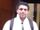## How do i print the last remaining in a string?

I have a string JOHN that contains 4 characters. I want to delete the 4th letter every time.
example:
JOHN- 4th letter is N. N is deleted.
Now the string is reduced to JOH
JOH- count 3 letters and go back to the beginning and count J as 4th letter. J is deleted.
JO- 4th letter is O. O is deleted.
last remaining letter is J which should be printed as the result.

how do I do this with sas?

7 REPLIES 7novinosrin
Tourmaline | Level 20

## Re: How do i print the last remaining in a string?

``````

data have;
str='JOHN';
run;

data want;
set have;
do until(lengthn(str)=1);
substr(str,lengthn(str),1)=' ';
output;
end;
run;``````
str
JOH
JO
Jnovinosrin
Tourmaline | Level 20

## Re: How do i print the last remaining in a string?

Must it be length of 4 as a start?

Then

``````
data have;
str='JOHNhhk';
run;

data want;
set have;
ln=4;
do until(ln=1);
substr(str,ln)=' ';
output;
ln=lengthn(str);
end;
run;
proc print noobs;run;``````

str ln
JOH 4
JO 3
J 2

## Re: How do i print the last remaining in a string?

Sorry. There’s a mistake in the question. Lets take a longer word. Say DONALD. There are 6 letters. SAS should count the 6th letter in DONALD in each iteration and delete the 6th letter.
DONALD -delete D
DONAL - delete D
ONAL - delete N
OAL - delete L
OA - delete A
Print the result as O

## Re: How do i print the last remaining in a string?

It's a bit late for me, so the brain is turning to mush here.  But here's an approach that should work.

If you have a six-letter word, you will always want the second letter.  So ...

Set up an array with 20 elements, all zero.

Apply some numeric algorithm that just escapes me for now.  But after 1 iteration, it is 6 and thus turns the sixth array element to 1.

Then repeat.  On the second iteration, the mystery algorithm generates 1, and thus turns the first array element to 1.

On the third iteration, turn the third array element to 1.

The point is that you don't need to change the character string.  You can work with the positions of the elements, and track which ones have already been changed.  When the number of changed elements is one less than the number of letters in the word, the first zero is the position of the letter that remains.

## Re: How do i print the last remaining in a string?

Hi @sneha_dodle,

Try this:

``````%let string=DONALD;

data _null_;
c="&string";
p=length(c);
do i=1 to p-1;
substr(c,mod(p-1,length(c))+1,1)=' ';
c=compress(c);
put c;
end;
run;``````

Edit: Not surprisingly, the sequence of the last remaining positions (of initial strings of length 1, 2, 3, ...) can be found in the OEIS: http://oeis.org/A128982.

## Re: How do i print the last remaining in a string?

Wow.  Didn't know there was such a thing as OEIS.  You not only knew about it, but knew how to find the right problem.

Maybe this is my fault for looking at this before the morning cup of coffee, but is that formula correct?  Isn't it looking for the 7th character on the first iteration?

## Re: How do i print the last remaining in a string?

Actually, I first wrote my program and then suspected that there might be a corresponding entry in the OEIS -- given that the resulting sequence of integers is both non-trivial and easily defined.

On the first iteration in the example the character at position mod(p-1,length(c))+1=mod(6-1,6)+1=5+1=6 is replaced with a blank, as it should.

Discussion stats
• 7 replies
• 551 views
• 4 likes
• 4 in conversation